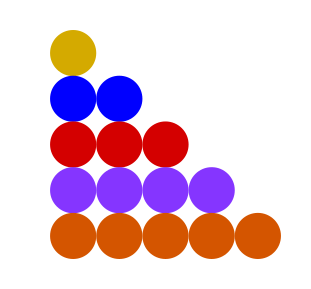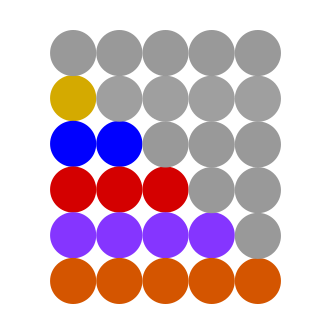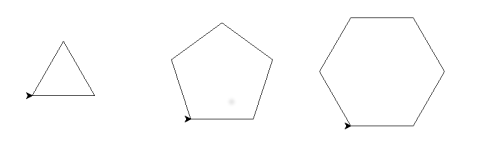# Recognising patterns

Martin McBride, 2016-12-22
Tags turtle graphics triangle numbers
Categories algorithms computational thinking

Whenever you tackle any kind of programming problem, you will probably notice patterns. You might see certain similarities between different cases, or maybe you will notice certain cases which never occur. You might see patterns in data, or you might notice a similarity between the problem you are trying to solve and another, totally different problem which already has a solution. Sometimes the pattern will be obvious, sometimes you need to look at things in a slightly different way before you see it.

Pattern recognitions is the most creative, and arguably the most important, part of computational thinking. It is often the first step in discovering a new algorithm. Here are some examples.

## Sum of natural numbers

We will start with a well known example from maths - what is the sum of the first n natural numbers? By natural numbers, we mean the numbers 1, 2, 3...

For example, when n = 5, the sum is:

1+2+3+4+5 = 15


What is the sum of the first 1,000,000 numbers? Well we could write a loop to calculate it, but that would a million calculations - a modern computer can do it in the blink of an eye, but it would still be nice to have a simple formula instead of having to loop. So lets calculate a few more cases, to look for a pattern:

n = 1: 1
n = 2: 1+2 = 3
n = 3: 1+2+3 = 6
n = 4: 1+2+3+4 = 10
n = 5: 1+2+3+4+5 = 15


This doesn't really help, so we could try a diagram. These are called the triangular numbers, as we can see from this picture:Now we might be on to something - the number of dots relates to the area of the triangle. But triangle areas are tricky things, usually involving trigonometry, and all we are trying to do here is count dots. Maybe redrawing our picture as a right angled triangle might help:Now we are getting somewhere! If we add some grey dots, we can make a rectangle:Notice that the number of grey dots is exactly the same as the number of coloured dots. The rectangle is 5 dots wide by 6 dots tall, so there are 30 dots altogether. The number of coloured dots is half that, 15 (the right answer for n=5, according to the table above).

In general the rectangle will be n dots wide by (n+1) dots tall, so the sum of all numbers up to n will be:

sum(n) = n*(n+1)/2


## Turtle graphics

In the section on decomposition we saw how to draw a square. What about other polygons?

Here we have drawn an equilateral triangle, and a regular pentagon and hexagon:If you remember, to draw a square we did the following:

• Square - repeat 4 times: forward(100), left(90)

To draw the other polygons we do

• Triangle - repeat 3 times: forward(100), left(120)
• Pentagon - repeat 5 times: forward(100), left(72)
• Hexagon - repeat 6 times: forward(100), left(60)

Where did the angles come from? Well let's assume we found them by trial and error. We now have a pattern to investigate - what links these pairs of numbers?

(3, 120) (4, 90) (5, 72) (6, 60)

The pattern is fairly simple - if you multiply each pair of numbers together, the result is 360 is each case.

This isn't so surprising. If you think about the turtle walking round each shape, turning at each corner, it will always end up facing in the same direction as when it started, so it must have turned 360 degrees in total. It is actually a well known mathematical result that the exterior angle of a regular polygon is 360 divided by the number of sides.

Just by looking at patterns, we have discovered a rule of geometry and also are well on the way to creating an algorithm to draw a regular polygon of any type using a turtle.

What happens if you choose an angle which doesn't divide into 360? For example, if we chose an angle of 108 degrees, the turtle draws 10 sides before it returns to its original position, and creates a shape with 10 corners. What is the pattern here?Copyright (c) Axlesoft Ltd 2021